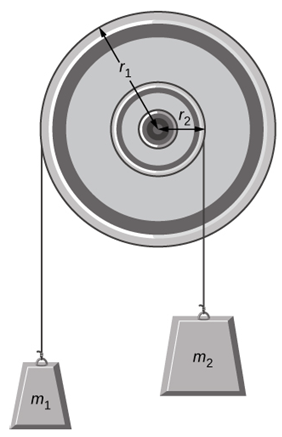# PhysicsFind accelerations- Angular and linear

#### Cbarker1

##### Active member
Dear Every Body,

I need some help. First question: Is the tension of the string is the same or different?
A pulley of moment of inertia 2.7 kg [FONT=&quot]·
m2 is mounted on a wall as shown in the following figure. Light strings are wrapped around two circumferences of the pulley and weights are attached. Assume the following data: r1 = 47 cm,
r2 = 20 cm,
m1 = 1.0 kg,
and m2 = 1.9 kg.
[/FONT](a)
What is the angular acceleration in rad/$s^2$ of the pulley?

(b)
What is the linear acceleration (in m/s2) of the weights?
a1

a2

#### Klaas van Aarsen

##### MHB Seeker
Staff member
Hi Cbarker1 ,

Which formulas do you have that:
- relate torque to angular acceleration?
- relate torque to force?

Can we apply them?

#### Cbarker1

##### Active member
Hi Cbarker1 ,

Which formulas do you have that:
- relate torque to angular acceleration?
- relate torque to force?

Can we apply them?
$\Sigma \tau=I\alpha$
$\tau=Fr$
$\Sigma F=ma$
For the first hanging mass:
$m_1g-T=m_1a_1$
$T=m_1g-m_1a_1$
For the second hanging mass:
$T=m_2a_2+m_2g$

#### Klaas van Aarsen

##### MHB Seeker
Staff member
$\Sigma \tau=I\alpha$
$\tau=Fr$
$\Sigma F=ma$
For the first hanging mass:
$m_1g-T=m_1a_1$
$T=m_1g-m_1a_1$
For the second hanging mass:
$T=m_2a_2+m_2g$
Good!
We also need that the speed of both masses match the speed that the wheel turns (where the masses are attached).
Can we also catch those in formulas?

#### Cbarker1

##### Active member
Do the mass have same tension?

#### Klaas van Aarsen

##### MHB Seeker
Staff member
Do the mass have same tension?
If the system were in equilibrium, the corresponding torques would have to cancel.
Since they are at different distances, that means that in equilibrium the tensions have to be different.
So we can't assume that the tensions are the same, and we have to keep them like $T_1$ and $T_2$ instead of just $T$.

#### Cbarker1

##### Active member
If the system were in equilibrium, the corresponding torques would have to cancel.
Since they are at different distances, that means that in equilibrium the tensions have to be different.
So we can't assume that the tensions are the same, and we have to keep them like $T_1$ and $T_2$ instead of just $T$.
$$T_2=1.9a_2+1.9g$$
$$T_1=g-a_1$$
$$T_1r_1+T_2r_2=I\alpha$$

#### Klaas van Aarsen

##### MHB Seeker
Staff member
$$T_2=1.9a_2+1.9g$$
$$T_1=g-a_1$$
$$T_1r_1+T_2r_2=I\alpha$$
Let's be careful with our signs.
The tensions that act on the masses both point upward yes?
Ideally we pick a direction up or down that we call positive and then stick to it.
How about picking 'up' to be 'positive' (it seems you already did)?

Which directions did you pick for $a_1$ and $a_2$?
Since we don't know yet if they are up or down, can we pick them both to be in the 'positive' direction?
If we do, there is a sign that we need to correct.

As for the angular acceleration, the torques oppose each other do they not?
That means one of them should have a minus sign.

Can we also include 2 more equations for the speeds of the masses that each must equal the speed of the wheel where the strings attach?

#### Cbarker1

##### Active member
I think $$\displaystyle a_1$$ should be positive and $$\displaystyle a_2$$ should be negative.

Last edited:

#### Klaas van Aarsen

##### MHB Seeker
Staff member
I think $$\displaystyle a_1$$ should be positive and $$\displaystyle a_2$$ should be negative.
That's also fine.
A drawing would be nice then, since otherwise I have no clue which vector points where.

#### Cbarker1

##### Active member
here is the picture